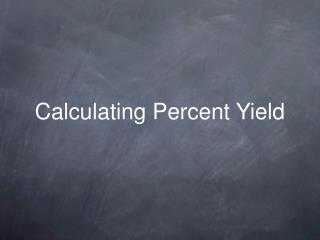DownloadDownload PresentationCalculating Percent Yield

# Calculating Percent Yield

Télécharger la présentation## Calculating Percent Yield

- - - - - - - - - - - - - - - - - - - - - - - - - - - E N D - - - - - - - - - - - - - - - - - - - - - - - - - - -
##### Presentation Transcript

1. Calculating Percent Yield

2. Introduction • The percent yield of a reaction is a measure of how efficient we were at running the reaction. • High percent yields (>95%) are excellent. • Low percent yields (>50%) are usually poor. • Most reactions have percent yields between 50% and 95%.

3. Introduction • The percent yield of a reaction is ... • ... the actual yield ... • ... divided by the theoretical yield ... • ... times 100%. actual yield × % yield = 100% theoretical yield

4. Introduction • In order to calculate % yield, we need to know ... • ... the actual yield (from experiment) • ... the theoretical yield (from calculation) • which is a stoichiometric calculation. actual yield × % yield = 100% theoretical yield

5. Chlorobenzene, C6H5Cl, is used in the production of many important chemicals, such as aspirin, dyes, and disinfectants. One industrial method of preparing chlorobenzene is to react benzene, C6H6, with chlorine, as represented by the following equation. C6H6(l) + Cl2(g) → C6H5Cl(s) + HCl(g) When 36.8 g of C6H6 react with an excess of Cl2, the actual yield of C6H5Cl is 38.8 g. What is the percent yield of C6H5Cl?

6. When 36.8 g of C6H6 react with an excess of Cl2, the actual yield of C6H5Cl is 38.8 g. What is the percent yield of C6H5Cl? M (g/mol) 78.1 70.9 112.6 36.45 C6H6(l) + Cl2(g) → C6H5Cl(s) + HCl(g) m (g) 36.8 38.8 n (mol) 0.471 0.471 mC6H6 36.8 g = = 0.471 mol nC6H6 = MC6H6 78.1 g/mol coeff C6H5Cl 1 = nC6H5Cl = nC6H6 = 0.471 mol 0.471 mol coeff C6H6 1 We need to find the moles of C6H6 we started with and the theoreticalyield of C6H5Cl. Next, we put in the masses we know. The first thing we need to do is to find the molar masses, M, of the reactants and products.

7. When 36.8 g of C6H6 react with an excess of Cl2, the actual yield of C6H5Cl is 38.8 g. What is the percent yield of C6H5Cl? M (g/mol) 78.1 70.9 112.6 36.45 C6H6(l) + Cl2(g) → C6H5Cl(s) + HCl(g) m (g) 36.8 38.8 n (mol) 0.471 0.471 mtheoretical 53.0 = mtheoretical = ntheoretical MC6H5Cl = (0.471 mol) (112.6 g/mol) 53.0 g Now we can find the mass of C6H5Cl that would have been formed if all of the C6H6 reacted with all of the Cl2.

8. When 36.8 g of C6H6 react with an excess of Cl2, the actual yield of C6H5Cl is 38.8 g. What is the percent yield of C6H5Cl? M (g/mol) 78.1 70.9 112.6 36.45 C6H6(l) + Cl2(g) → C6H5Cl(s) + HCl(g) m (g) 36.8 38.8 n (mol) 0.471 0.471 mtheoretical 53.0 mactual 38.8 g = × × percent yield = 100% = 100% 73.2% mtheoretical 53.0 g Finally, we have enough information to calculate the percent yield.